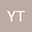Miscellaneous Reverse Order Laws for Generalized Inverses of Matrix Products with Applications
•• Yongge Tian
Yongge TianOne of the fundamental research problems in the theory of generalized inverses of matrices is to establish reverse order laws for generalized inverses of matrix products. Under the assumption that $A$, $B$, and $C$ singular matrices of the appropriate sizes, two reverse order laws for generalized inverses of the matrix products $AB$ and $ABC$ can be written as $(AB)^{(i,\ldots,j)} = B^{(i_2,\ldots,j_2)}A^{(i_1,\ldots,j_1)}$ and $(ABC)^{(i,\ldots,j)} = C^{(i_3,\ldots,j_3)}B^{(i_2,\ldots,j_2)}A^{(i_1,\ldots,j_1)}$, or other mixed reverse order laws. These equalities do not necessarily hold for different choices of generalized inverses of the matrices. Thus it is a tremendous work to classify and derive necessary and sufficient conditions for the reverse order law to hold because there are all 15 types of $\{i,\ldots, j\}$-generalized inverse for a given matrix according to combinatoric choices of the four Penrose equations. In this paper, we shall establish several decades of mixed reverse order laws for $\{1\}$- and $\{1,2\}$-generalized inverses of $AB$ and $ABC$, and give a classified investigation to a family of reverse order laws $(ABC)^{(i,\ldots,j)} = C^{-1}B^{(k,\ldots,l)}A^{-1}$ for the eight commonly-used types of generalized inverses by means of the block matrix representation method (BMRM) and the matrix rank method (MRM). A variety of consequences and applications these reverse order laws are presented.